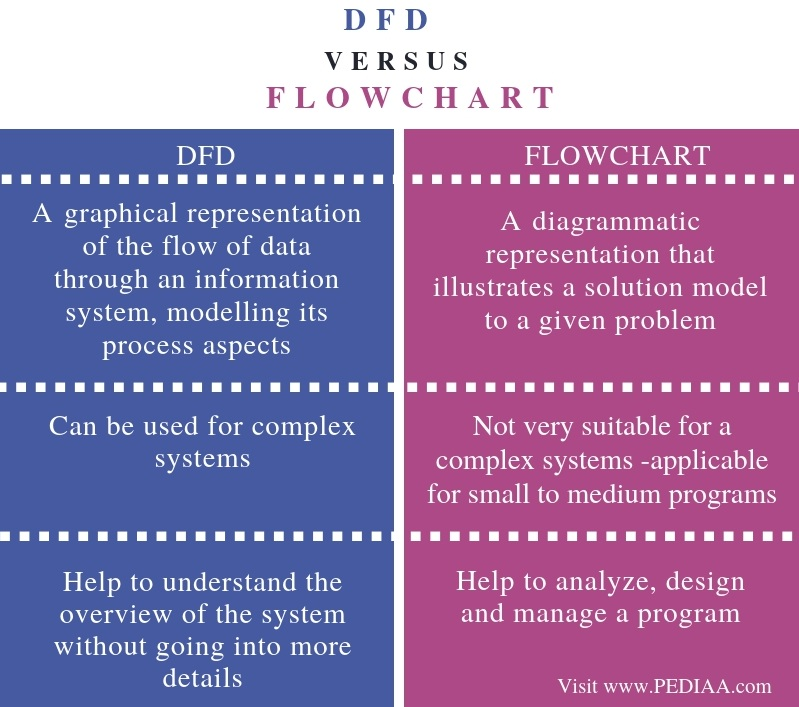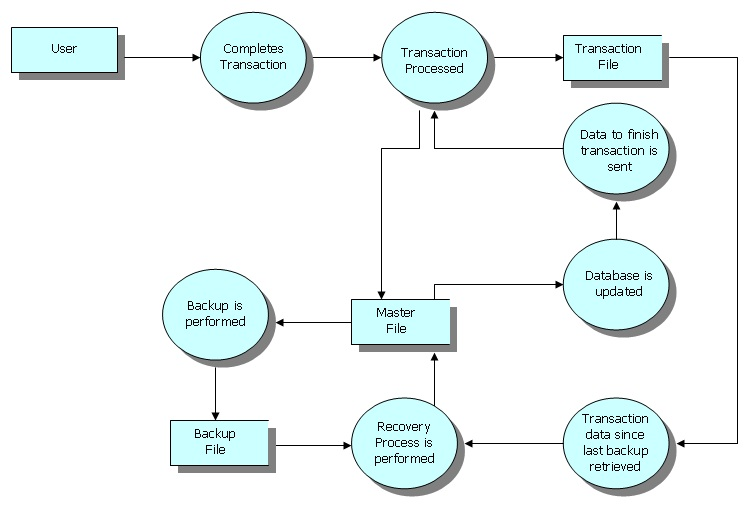# What is the Difference Between DFD and Flowchart

The main difference between DFD and Flowchart is that DFD is a graphical diagram that represents the data flow of a system while flowchart is a graphical diagram that represents the sequence of steps to solve a problem.

Developing software is a complex process, and it is difficult to write the program of the entire system directly. Therefore, it is necessary to model the system to get an understanding and then to program the modules. There are various diagrams that help to model the system. DFD and flowchart are two of them. DFD illustrates the inputs, outputs, how the data flows through the system and where data will be stored. On the other hand, a flowchart helps to illustrate the steps to solve the problem. It can be used as the basis to write the program.

### Key Areas Covered

1. What is DFD
– Definition, Functionality
2. What is a Flowchart
– Definition, Functionality
3. What is the Difference Between DFD and Flowchart
– Comparison of Key Differences

### Key Terms

Data Flow Diagram or DFD, Flowchart## What is DFD

DFD stands for Data Flow Diagram. It represents how a system processes data and describes where the data comes from, where it goes and how the data is stored.  DFD became popular during the year 1970. There are two types of notations for DFD. They are the Yourdon and Coad, Gane and Sarson.

Youdon and Coad– This type of diagrams are used for system analysis and design. Circles represent the processes.

Gane and Sarson – This type of diagrams are used for information systems. Square with round corners represent the processes.Some common DFD notations are as follows.

Process – Transforms incoming data flow into the outgoing data flow

Data Store – Represents the repositories of data in the system

Data Flows – Represents the pathway of data flow

External Entities – Represents the objects outside the system. The system communicates with these external entities. They are the sources and destinations of the system’s inputs and outputs.

Furthermore, DFD diagrams have layers or levels to organize data. The context Diagram is the top level. It generalizes the functionality of the entire system and relationship with the external entities. Level 1 Diagram provides more details than the context diagram. However, level 2 diagrams provide more details than level 1, and Level 3 diagrams provide more details than level 2 diagrams. Furthermore, it is necessary to break down the processes until reaching the pseudo code, which is a human-readable structure to understand the program.

## What is a Flowchart

A flowchart is a diagram that helps to represent an algorithm. In other words, a flowchart helps to write down an algorithm.

### Algorithm

In computing, an algorithm is a step by step procedure to solve a given problem. If the problem is complex, it is possible to divide the problem into multiple subproblems and solve each of them to solve the main problem.

An algorithm to find the area of the rectangle is as follows.

1. Initialize area = 0
2. Enter length and width
3. Multiply them and store the result in area
4. Print area.

The flowchart diagram to calculate area is as follows.Figure 1: A Flowchart

Therefore, a flowchart is a graphical representation of writing an algorithm. Furthermore, the notations of flowchart symbols are as follows.

Oval – Represents the start and the termination

Rhombus – Represents input and output operations (Inputs can be user inputs while outputs are the results displayed on the screen)

Rectangle – Represents a process. E.g. – variable initialization, calculations

Diamond symbol – Used for decision making

Circle (small) – It is connectors

Arrow – Represent the sequence of steps

## Difference Between DFD and Flowchart

### Definition

DFD is a graphical representation of the flow of data through an information system, modelling its process aspects. In contrast, a flowchart is a diagrammatic representation that illustrates a solution model to a given problem. Thus, this explains the basic difference between DFD and flowchart.

### Applicability

DFD can be used for complex systems. Although a flowchart is not very suitable for a complex system, it is applicable for small to medium programs. Hence, this is another difference between DFD and flowchart.

### Usage

Moreover, their usage attributes to another difference between DFD and flowchart is their usage. DFDs help to understand the overview of the system without going into more details while flowcharts help to analyze, design and manage a program.

### Conclusion

In summary, DFD and Flowchart are two diagram types that help to develop software. The main difference between DFD and Flowchart is that DFD is a graphical diagram that represents the data flow of a system while a flowchart is a graphical diagram that represents the sequence of steps to solve a problem.

##### Reference:

1. What Is DFD? Data Flow Diagram Symbols and More, SmartDraw, 7 Sept. 2018, Available here.
2. “Data Flow Diagram.” Wikipedia, Wikimedia Foundation, 30 Oct. 2018, Available here.
3. “Flowchart.” Wikipedia, Wikimedia Foundation, 18 Oct. 2018, Available here.

##### Image Courtesy:

1. “Backup-DFD” By Sukari at English Wikipedia – Created by Sukari at English Wikipedia (Public Domain) via Commons Wikimedia